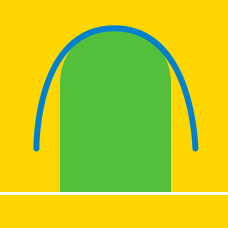Calculus

# Area between Curve and x-axis

Find the area of the region bounded by $y \geq x^2 - 25$ and $y \leq 0$.

What is the area of the region bounded by the curve $y= 9{x}^2-54x$ and the $x$-axis ?

What is area of the region bounded by the curve $y = \sqrt{x}$ and the $x$-axis in the interval $0 \leq x \leq 4?$Consider the region bounded by the curve $f(x)= x(x-b)(x-a)$ and the $x$-axis, where $b=5$ and $a > 5$. If the bounded region above the $x$-axis and the bounded region below the $x$-axis have the same area, what is the value of $a ?$

Let $C$ be the curve obtained by a parallel translation of the curve $y=a{x}^2$ $(a>0)$ by $4$ units in the positive direction of the $x$-axis. If the area of the region bounded by the curve $y=a{x}^2 ,$ the curve $C,$ and the $x$-axis is $16,$ what is $a?$

×